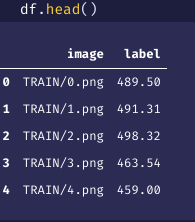# CNN network is not learning (losses above the million)

Hi there,
I’m trying to create a CNN network to predict a single float number from an image.

The float output can assume the values from 0.0 to 20000.0.
Each image is a coloured 7x7 image, labelled with a float number as well.

This is my DataBlock

``````x_column = "image"
y_column = "label"

dls = DataBlock(
blocks=(ImageBlock, RegressionBlock),
get_x=ColReader(x_column, pref='', suff=''),
get_y=ColReader(y_column),
batch_tfms=[]
).dataloaders(df)
``````

df is like thatthe CNN is

``````def conv(ni, nf, ks=3, act=True):
res = Conv2d(ni, nf, stride=2, kernel_size=ks, padding=ks//2)
if act: res = Sequential(res, ReLU())
return res

simple_cnn = Sequential(
conv(3, 12, ks=5),
conv(12, 24),
conv(24, 48),
conv(48, 1, act=False),
Flatten(),
)
``````

and the learner is

``````learn = Learner(dls, simple_cnn, loss_func=MSELossFlat(), cbs=ActivationStats(with_hist=True))
``````

very simple, but the network isn’t learning, even if use smaller learning rates the result is the same

any help?
is the correct model for predicting a number from an image, even if the network never saw the number in the training labels set?

thanks

Can you explain a little more what the images are? How do these images map to the Y value?

Hello Kevin,
these are gramian angular field images, each one is created from a [7, 3] matrix.
The matrix represents a time-series, each column is a step, in this case, 7 steps,
and the rows are the features, 3.

For example, I transform this matrix
[[ 1.2, 2.4, 3.1], [1.9, 1.8, 3.4], [2.3, 3.4, 3.4], [2.3, 4.5, 5.5], [4.5, 1.9, 3.4], [1.2, 2.4, 3.1], [1.7, 2.4, 5.6]]

into this imageand I label it with 5.4 because in the timeseries, in the 8th step, one of the 3 features contains the 5.4 number

1 Like

Ok, thanks for the explanation. Is this dataset easy to regenerate or would there be a way to create a sample notebook gist? I can look into it if you can get me something to play around with. I don’t see the issue off the top of my head, but I would look at running one batch through the model and loss function and watch what it’s doing. So I would start with grabbing a single data batch using `x,y = dls.one_batch()`

and then pass x through your model.

`out = simple_cnn(x)`

then try passing `y` and `out` into your MSELossFlat() function and see what the numbers look like.

I would add `BatchNorm` to this, and in what arange are the input values?
I also use this loss for regression when the target values are high:

``````def ScaledMSE(scale=1000):
def _inner(inp, tar): return MSELossFlat()(inp, tar)/scale
return _inner
``````

An other trick that works great for regression is discrtizing y values in buckets:
[0,100,200,300,…] and using CrossEntropy instead of MSE.

1 Like